# Chapter 10:Deflections of beams¶

## Example 10.1 page number 501¶

In :
#Given
dia = 400   #mm - The diameter of a pulley
E = 200     #Gpa - Youngs modulus
t = 0.6     #mm - The thickness of band
c = t/2     #mm - The maximum stress is seen
#Caliculations

stress_max = E*c*(10**3)/(dia/2) #Mpa - The maximum stress on the crossection occurs at the ends
print "The maximum bending stress developed in the saw ",stress_max,"Mpa"

The maximum bending stress developed in the saw  300.0 Mpa


## Example 10.3 page number 512¶

In :
#Given
import numpy
l_ab = 1.0   #L in - The length of the beam
F_D = 1.0    #W lb/in - The force distribution
F = F_D*l_ab #WL - The force applied
#Beause of symmetry the moment caliculations can be neglected
#F_Y = 0
R_A = F/2 #wl - The reactive force at A
R_B = F/2 #wl - The reactive force at B
#EI - The flxure rigidity is constant and 1/EI =1 # k

#part - A
#section 1--1
l_1 = [0,0.1,0.2,0.3,0.4,0.5,0.6,0.7,0.8,0.9,1] #L taking each section at 0.1L distance
M_1 = [0,0,0,0,0,0,0,0,0,0,0]
v = [0,0,0,0,0,0,0,0,0,0,0]
for i in range(10):
v[i] = R_A - F_D*l_1[i]
M_1[i] = R_A*l_1[i] - F_D*(l_1[i]**2)/2
# (EI)y'' = M_1[i] we will integrate M_1[i] twice where variable is l_1[i]
#(EI)y'-

M_1_intg1 = R_A*(l_1[i]**2)/4 - F_D*(l_1[i]**3)/6 - F_D*(l_ab**3)*l_1[i]/24 #integration of x**n = x**n+1/n+1
#(EI)y- Using end conditions for caliculating constants

M_1_intg2 = R_A*(l_1[i]**3)/12.0 - F_D*(l_1[i]**4)/24.0 + F_D*(l_ab**3)*l_1[i]/24.0
#Equations

l_1 = [0,0.1,0.2,0.3,0.4,0.5,0.6,0.7,0.8,0.9,1] #L taking each section at 0.1L distance
M_1_intg2 = [0,0,0,0,0,0,0,0,0,0,0]
Y = [0,0,0,0,0,0,0,0,0,0,0]
for i in range(10):
M_1_intg2[i] = (l_1[i]**3)/12.0 - (l_1[i]**4)/24.0 - l_1[i]/24.0   # discluding every term for ruling out float values
Y[i] = M_1_intg2[i] #W(l**4)/EI  k = 1/EI
#The precision is very less while caliculating through this equation because the least count in X direction is 0.1
print "a) The maximum displacement in y direction is",min(Y),"W(l**4)/EI "
print "a) The maximum deflection occured at",l_1[Y.index(min(Y))],"L"

#Part - B
#Graphs
import numpy as np
values = M_1
y = np.array(values)
t = np.linspace(0,1,11)
poly_coeff = np.polyfit(t, y, 2)
import matplotlib.pyplot as plt
%matplotlib inline
plt.plot(t, y, 'o')
plt.plot(t, np.poly1d(poly_coeff)(t), '-')
plt.show()
print "b) The above graph is bending moment graph"
values = Y
y = np.array(values)
t = np.linspace(0,1,11)
poly_coeff = np.polyfit(t, y, 2)
import matplotlib.pyplot as plt
plt.plot(t, y, 'o')
plt.plot(t, np.poly1d(poly_coeff)(t), '-')
plt.show()
print "b)The above graph is beam displacement graph"
print "b)The maximum occures in the middle from the above graph  "

a) The maximum displacement in y direction is -0.0130208333333 W(l**4)/EI
a) The maximum deflection occured at 0.5 L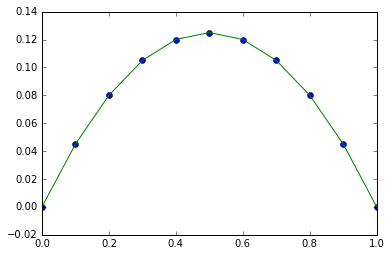b) The above graph is bending moment graph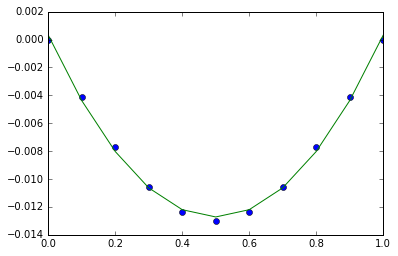b)The above graph is beam displacement graph
b)The maximum occures in the middle from the above graph


## Example 10.5 page number 517¶

In :
#Given
#because of symmetry the problem can be solved by considering first half
#Given
import numpy

l_ab = 1.0       #L in - The length of the beam
F_D = 1.0        #W lb/in - The force distribution
F = F_D*l_ab     #WL - The force applied
#Beause of symmetry the moment caliculations can be neglected
#EI - The flxure rigidity is constant and 1/EI =1 # k

#part - A
#section 1--1
l_1 = [0,0.1,0.2,0.3,0.4,0.5,0.6,0.7,0.8,0.9,1] #L taking each section at 0.1L distance
M_1 = [0,0,0,0,0,0,0,0,0,0,0]
v = [0,0,0,0,0,0,0,0,0,0,0]

# M_1_intg2 = 0, the displacement at the end of rod is 0 since its rigid
R_A =  (F_D*(l_1**4)/24.0 + F_D*(l_ab**3)*l_1/48.0)/((l_1**3)/6.0)
R_C = R_A #WL - symmetry
R_B = 1-R_A # WL - F_Y = 0, the equilibrium in Y direction
print "The reaction at A is",R_A ,"WL"
print "The reaction at B is",R_B ,"WL"
print "The reaction at C is",R_C ,"WL"

The reaction at A is 0.375 WL
The reaction at B is 0.625 WL
The reaction at C is 0.375 WL


## Example 10.7 page number 521¶

In :
import warnings
warnings.filterwarnings('ignore')
import numpy as np
%matplotlib inline
import matplotlib.pyplot as plt

l_ac = 5 #m - The length of the beam
l_ab = 4 #m - The length of ac on beam
l_bc = 1 #m - The length of bc on beam
F = 20 #N - force applied on beam at 'b'
R_A = F/2    #wl - The reactive force at A
R_B = F/2    #wl - The reactive force at B
I_ab = 4 #I The moment of inertia of part AB
I_bc = 1 #I - The momemt of inertia of part BC
R_A = F*(l_bc/l_ac) #N- The reaction at joint A
R_B = F*(l_ab/l_ac) #N- The reaction at joint B
E = 1 #E youngs modulus

#0<x<4
x = [0,1,2,3,4]
M = [0,0,0,0,0]
y = [0,0,0,0,0]
for i in range(5):
M[i] = 4*x[i] #integration of x**n = x**n+1/n+1
#y_2[i] = 4*x[i]/(E*I_ab) #The
#y_1[i] = 4*(x[i]**2)/(E*I_ab) -4.8/(E*I_bc)  #The constant can be found by conditions y(o) = y(c) = 0
y[i]  =   4*(x[i]**2)/(6*E*I_ab)  -4.8*x[i]/(E*I_bc)  #elastic curve constant can be found by Y_1(0) = 0

#0<x_1<1
x_1 = [4,5]
m = [0,0]
Y = [0,0]
for i in range(2):
m[i] = 16 - 16*x_1[i]    #integration of x**n = x**n+1/n+1
# Y_2 = (16 - 16*x_1[i])/(E*I_ab)
#Y_1 = (16*x_1[i]-8*(x_1[i]**2) +8 - 4.8)/(E*I_ab)#The constant can be found by conditions y(o) = y(c) = 0
Y[i] =  (8*(x_1[i]**2)-8*(x_1[i]**3)/3 +(8-4.8)*x_1[i] - 4*4.8 )/(E*I_ab) #elastic curve constant can be found by Y_1(0) = 0

#Graphs
values = y
y = np.array(values)
t = np.linspace(0,1,5)
poly_coeff = np.polyfit(t, y, 2)
plt.plot(t, y, 'o')
plt.plot(t, np.poly1d(poly_coeff)(t), '-')
plt.show()
print "b) The shape from x belongs to 0<x<4"
values = Y
y = np.array(values)
t = np.linspace(0,1,2)
poly_coeff = np.polyfit(t, y, 2)
plt.plot(t, y, 'o')
plt.plot(t, np.poly1d(poly_coeff)(t), '-')
plt.show()
print "b) The shape from x belongs to 4<x<5"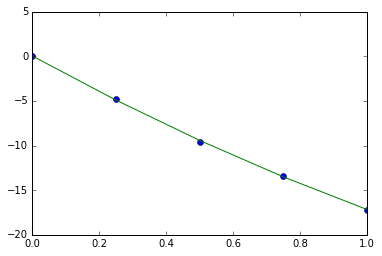b) The shape from x belongs to 0<x<4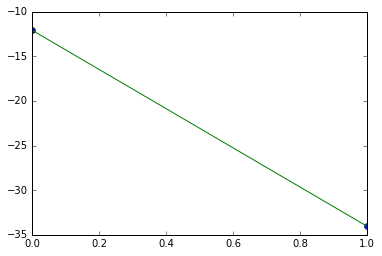b) The shape from x belongs to 4<x<5


## Example 10.10 page number 529¶

In :
k = 24.0*(10**12)    #N.mm2 Flexure rigidity
E = 200.0            #Gpa - Youngs modulus of the string
l = 5000.0           #mm - The length of the string
C_A = 300.0          #mm2 - crossection area
P = 50.0             #KN - The force applies at the end
a = 2000.0           #mm - The distance C-F
x = 1#X - let it be a variable X
y_d = x*(a**3)/(3*k)                                 #Xmm The displacement at D, lets keep the variable in units part
y_p  = -P*(10**3)*(16*(a**3)-12*(a**3)+(a**3))/(k*6) #mm The displacement due to p
e_rod = l/(C_A*E*(10**3))                            #Xmm -deflection, The varible is in units
e_rod
X = y_p/(2*e_rod+y_d)  # By equating deflections
y_d_1 = X*(a**3)/(3*k) # the deflection of point D
print "The deflection of point D",round(y_d_1,2),"mm"

The deflection of point D -5.56 mm


## Example 10.11 page number 530¶

In :
import math
l = 15          #in - The length of the crossection
b = 33.9        #in - the width of the crossection
L = 100         #in The length of the cantilever
E = 29*(10**6)  #psi The youngs modulus of the material used
I_Z = 315       #in4 - the moment of inertia wrt Z axis
I_y = 8.13      #in4 - the moment of inertia wrt Y axis
o = 5           # degrees - the angle of acting force
P = 2000        #k the acting force
P_h = P*math.sin(math.radians(o)) #k - The horizantal component of P
P_v = P*math.cos(math.radians(o)) #k - The vertical  component of P
e_h =  P_h*(L**3)/(3*E*I_y)       # the horizantal component of deflection
e_v =  P_v*(L**3)/(3*E*I_Z )      # the vertical component of deflection
e = pow((e_h**2 + e_v**2),0.5)
print "the horizantal component of deflection",round(e_h,3) ,"in"
print "the vertical component of deflection",round(e_v,3) ,"in"
print "the resultant deflection",round(e,3) ,"in"

the horizantal component of deflection 0.246 in
the vertical component of deflection 0.073 in
the resultant deflection 0.257 in


## Example 10.13 page number 533¶

In :
#Given
l = 50.0           #mm - The length of the crossection
b = 50.0           #mm - the width of the crossection
m = 15.3           # mass of the falling body
h = 75.0           #mm - The height of the falling body
p = m*9.81         #N the force acted due to the body
L = 1000.0         #mm The length of the cantilever
E = 200  #Gpa The youngs modulus of the material used
I = (l**4)/12 #mm - the moment of inertia
k = 300 #N/mm -the stiffness of the spring
#Rigid supports
e = m*9.81*(L**3)*(10**-3)/(48*E*I) #mm - the deflection of beam
imp_fact_a = 1 +pow((1 +2*h/e),0.5) #no units , impact factor
#spring supports
e_spr = h/k #mm the elongation due to spring
e_total = e_spr + e
imp_fact_b =  1 +pow((1 +2*h/e_total),0.5) #no units , impact factor
print "a) The maximum deflection when the beam is on rigid supports",round(e,3),"mm with impact factor",round(imp_fact_a ,2),
print "b) The maximum deflection when the beam is on spring supports",round(e_total,2),"mm with impact factor",round(imp_fact_b,2) ,

a) The maximum deflection when the beam is on rigid supports 0.03 mm with impact factor 71.7 b) The maximum deflection when the beam is on spring supports 0.28 mm with impact factor 24.17


## Example 10.15 page number 536¶

In :
#Given
E = 30*(10**3) #ksi - The youngs modulus of the material
stress_y = 40 #Ksi - yield stress
stress_max = 24.2 #Ksi - the maximum stress
l = 2          #in - The length of the crossection
b = 3       #in - the width of the crossection
h = 3 #in - the depth of the crossection
#lets check ultimate capacity for a 2 in deep section
M_ul = stress_y*b*(l**2)/4 #K-in the ultimate capacity
curvature = 2*stress_y/(E*(h/2) ) #in*-1 the curvature of the beam
curvature_max = stress_max/(E*(h/2)) #in*-1 The maximum curvature
print "the ultimate capacity",M_ul,"K-in"
print "the ultimate curvature",curvature_max,"in*-1"

the ultimate capacity 120 K-in
the ultimate curvature 0.000806666666667 in*-1


## Example 10.16 page number 543¶

In :
#Given
l_ad = 1600 #mm - The total length of the beam
l_ab = 600  #mm - The length of AB
l_bc = 600  #mm - The length of BC
e_1 = 0.24  #mm - deflection
e_2 = 0.48  #mm - deflection
E = 35      #Gpa
#Caliculation

A_afe = -(l_ab+l_bc)*e_1*(10**-3)/(2*E)
A_afe = -(l_ab)*e_2*(10**-3)/(4*E)
y_1_b = A_afe + A_afe #rad the slope at the tip  B
x_1 = 1200            #com from B
x_2 = 800             #com from B
y_b = A_afe*x_1 + A_afe*x_2 #mm The maximum deflection at tip B
print"The maximum deflection at tip B",round(y_b,2),"mm"
print "The slope at the tip  B",round(y_1_b,2) ,"radians"

The maximum deflection at tip B -4.11 mm
The slope at the tip  B -0.0 radians


## Example 10.19 page number 547¶

In :
#Given
import numpy
l_ab = 1.0   #L in - The length of the beam
F_D = 1.0    #W lb/in - The force distribution
F = F_D*l_ab #WL - The force applied
#Beause of symmetry the moment caliculations can be neglected
#F_Y = 0
R_A = F/2 #wl - The reactive force at A
R_B = F/2 #wl - The reactive force at B
#EI - The flxure rigidity is constant and 1/EI =1 # k

#part - A
#section 1--1
l_1 = [0,0.1,0.2,0.3,0.4,0.5,0.6,0.7,0.8,0.9,1] #L taking each section at 0.1L distance
M_1 = [0,0,0,0,0,0,0,0,0,0,0]
v = [0,0,0,0,0,0,0,0,0,0,0]
for i in range(10):
v[i] = R_A - F_D*l_1[i]
M_1[i] = R_A*l_1[i] - F_D*(l_1[i]**2)/2
# (EI)y'' = M_1[i] we will integrate M_1[i] twice where variable is l_1[i]
#(EI)y'-

M_1_intg1 = R_A*(l_1[i]**2)/4 - F_D*(l_1[i]**3)/6 - F_D*(l_ab**3)*l_1[i]/24 #deflection integration of x**n = x**n+1/n+1
#(EI)y- Using end conditions for caliculating constants

M_1_intg2 = R_A*(l_1[i]**3)/12.0 - F_D*(l_1[i]**4)/24.0 + F_D*(l_ab**3)*l_1[i]/24.0
#Equations

l_1 = [0,0.1,0.2,0.3,0.4,0.5,0.6,0.7,0.8,0.9,1] #L taking each section at 0.1L distance
M_1_intg2 = [0,0,0,0,0,0,0,0,0,0,0]
Y = [0,0,0,0,0,0,0,0,0,0,0]
for i in range(10):
M_1_intg2[i] = (l_1[i]**3)/12.0 - (l_1[i]**4)/24.0 - l_1[i]/24.0   # discluding every term for ruling out float values
Y[i] = M_1_intg2[i] #W(l**4)/EI  k = 1/EI
#The precision is very less while caliculating through this equation because the least count in X direction is 0.1
print " The maximum displacement in y direction is",min(Y),"W(l**4)/EI "
print " The maximum deflection occured at",l_1[Y.index(min(Y))],"L"

l_1 = [0,0.1,0.2,0.3,0.4,0.5,0.6,0.7,0.8,0.9,1] #L taking each section at 0.1L distance
M_1_intg1 = [0,0,0,0,0,0,0,0,0,0,0]
Y = [0,0,0,0,0,0,0,0,0,0,0]
for i in range(10):
M_1_intg1[i] = R_A*(l_1[i]**2)/4 - F_D*(l_1[i]**3)/6 - F_D*(l_ab**3)*l_1[i]/24
print " The maximum deflection is",min(M_1_intg1 ),"W(l**3)/EI "

 The maximum displacement in y direction is -0.0130208333333 W(l**4)/EI
The maximum deflection occured at 0.5 L
The maximum deflection is -0.05775 W(l**3)/EI


## Example 10.23 page number 554¶

In :
import numpy
l_ab = 1.0   #L in - The length of the beam
F_D = 1.0    #W lb/in - The force distribution
F = F_D*l_ab #WL - The force applied
#Beause of symmetry the moment caliculations can be neglected
#F_Y = 0
R_A = F/2    #wl - The reactive force at A
R_B = F/2    #wl - The reactive force at B
#EI - The flxure rigidity is constant and 1/EI =1 # k
#M_A and M_B are applied at the ends

#part - A
#section 1--1
l_1 = [0,0.1,0.2,0.3,0.4,0.5,0.6,0.7,0.8,0.9,1]    #L taking each section at 0.1L distance
M = [0,0,0,0,0,0,0,0,0,0,0]
for i in range(10):
M[i] = l_1[i]/2.0 - (l_1[i]**2)/2.0 -1.0/12.0    #The moment euation at 1--1 section
# M_1 = R_A*l_1[i]/2.0 - F_D*(l_1[i]**2)/2.0 -F_D*(l_ab**2)/12.0  #The moment euation at 1--1 section
# (EI)y'' = M_1[i] we will integrate M_1[i] twice where variable is l_1[i]
#(EI)y'
M_1_intg1 = R_A*(l_1[i]**2)/4 - F_D*(l_1[i]**3)/6 - F_D*(l_ab**2)*l_1[i]/12.0 #integration of x**n = x**n+1/n+1
#(EI)y

print "The moment at the end is ",M,"wl**2"

The moment at the end is  -0.0833333333333 wl**2


## Example 10.25 pagenumber 556¶

In :
import matplotlib.pyplot as plt
%matplotlib inline
import numpy as np
# This problem is divided into two parts
#Part _1
#Given

l = 1.0 #l - The length of the beam
p = 1.0 #W - The total load applied
#since it is triangular distribution
l_com = 0.66*l #l - The distance of force of action from one end
#F_Y = 0
#R_A + R_B = p
#M_a = 0 Implies that R_B = 2*R_A
R_A = p/3.0
R_B = 2.0*p/3

#Taking Many sections

#Section 1----1
l = [0,0.1,0.2,0.3,0.4,0.5,0.6,0.7,0.8,0.9,1] #L taking each section at 0.1L distance
M = [0,0,0,0,0,0,0,0,0,0,0]
v = [0,0,0,0,0,0,0,0,0,0,0]
for i in range(10):
v[i] = p*(l[i]**2) - p/3.0
M[i] = p*(l[i]**3)/(3.0)- p*l[i]/3.0

v = R_B #again concluded Because the value is tearing of

#Graph
values = M
y = np.array(values)
t = np.linspace(0,1,11)
poly_coeff = np.polyfit(t, y, 2)
plt.plot(t, y, 'o')
plt.plot(t, np.poly1d(poly_coeff)(t), '-')
plt.show()
values = v
y = np.array(values)
t = np.linspace(0,1,11)
poly_coeff = np.polyfit(t, y, 2)

plt.plot(t, y, 'o')
plt.plot(t, np.poly1d(poly_coeff)(t), '-')
plt.show()

#part B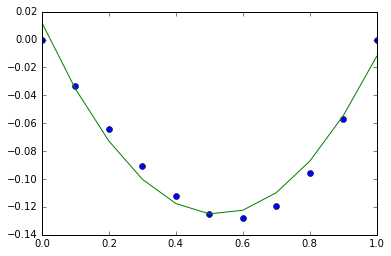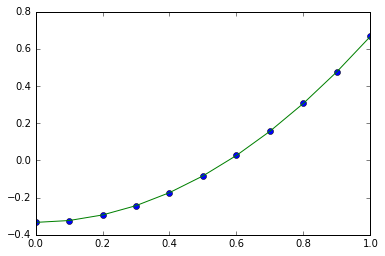In [ ]: